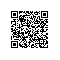# STL中的常用的vector，map，set，sort, list用法笔记 .

C++的标准模板库（Standard Template Library，简称STL）是一个容器和算法的类库。容器往往包含同一类型的数据。STL中比较常用的容器是vector，set和map，比较常用的算法有Sort等。
.

1.声明：
一个vector类似于一个动态的一维数组。
vector<int> a; //声明一个元素为int类型的vector a
vectot<MyType> a; //声明一个元素为MyType类型的vector a
这里的声明的a包含0个元素，既a.size()的值为0，但它是动态的，其大小会随着数据的插入

和删除改变而改变。
vector<int> a(100, 0); //这里声明的是一已经个存放了100个0的整数vector
2.向量操作

size_t size();             // 返回vector的大小，即包含的元素个数
void pop_back();           // 删除vector末尾的元素，vector大小相应减一
void push_back();          //用于在vector的末尾添加元素
T back();                  // 返回vector末尾的元素
void clear();              // 将vector清空，vector大小变为0

cout<<a<<endl;
cout<<a.at(5)<<endl;

int         intarray;
vector<int>         first_vector(intarray,         intarray         +         10);
vector<int>         second_vector(first_vector.begin(),first_vector.end());
class         man
{
public:
AnsiStirng         id;
AnsiString         mc;
}
vector<man>         manList;
man         thisman;
thisman.id="2001";
thisman.name="yourname";
manList.push_back         thisman;           //加入第一个元素
thisman.id="2002";
thisman.name="myname";
manList.push_back         thisman;           //加入第二个元素

manList.clear();           //清空
3.遍历
(1).      for(vector<datatype>::iterator it=a.begin(); it!=a.end();it++)
cout<<*it<<endl;
(2).     for(int i=0;i<a.size;i++)
cout<<a[i]<<endl;

Map是STL的一个关联容器，它提供一对一（其中第一个可以称为关键字，每个关键字只能在map中出现一次，第二个可能称为该关键字的值）的数据处理能力，由于这个特性
map内部的实现自建一颗红黑树(一种非严格意义上的平衡二叉树)，这颗树具有对数据自动排序的功能。

1.            声明方式：
Map<int, string> mapStudent;
2.            数据的插入

Map<int, string> mapStudent;
mapStudent.insert(pair<int, string>(1, “student_one”));

Map<int, string> mapStudent;
mapStudent.insert(map<int, string>::value_type (1, “student_one”));

Map<int, string> mapStudent;
mapStudent =       “student_one”;
mapStudent = “student_two”;
3.            map的大小

Int nSize = mapStudent.size();
4.            数据的遍历

map<int, string>::iterator iter;
for(iter = mapStudent.begin(); iter != mapStudent.end(); iter++)
Cout<<iter->first<<”        ”<<iter->second<<end;

map<int, string>::reverse_iterator iter;
for(iter = mapStudent.rbegin(); iter != mapStudent.rend(); iter++)
Cout<<iter->first<<”        ”<<iter->second<<end;

int nSize = mapStudent.size()
for(int nIndex = 1; nIndex <= nSize; nIndex++)
Cout<<mapStudent[nIndex]<<end;
5.            数据的查找（包括判定这个关键字是否在map中出现）

Int main()
{
Map<int, string> mapStudent;
mapStudent.insert(pair<int, string>(1, “student_one”));
mapStudent.insert(pair<int, string>(2, “student_two”));
mapStudent.insert(pair<int, string>(3, “student_three”));
map<int, string>::iterator iter;
iter = mapStudent.find(1);
if(iter != mapStudent.end())
{
Cout<<”Find, the value is ”<<iter->second<<endl;
}
Else
{
Cout<<”Do not Find”<<endl;
}
}

Lower_bound函数用法，这个函数用来返回要查找关键字的下界(是一个迭代器)
Upper_bound函数用法，这个函数用来返回要查找关键字的上界(是一个迭代器)

Equal_range函数返回一个pair，pair里面第一个变量是Lower_bound返回的迭代器，pair里面第二个迭代器是Upper_bound返回的迭代器，如果这两个迭代器相等的话，则说明map中不出现这个关键字，程序说明
mapPair = mapStudent.equal_range(2);
if(mapPair.first == mapPair.second)

cout<<”Do not Find”<<endl;
6.            数据的清空与判空

7.            数据的删除

iter = mapStudent.find(1);
mapStudent.erase(iter);

Int n = mapStudent.erase(1);//如果删除了会返回1，否则返回0

一下代码把整个map清空
mapStudent.earse(mapStudent.begin(), mapStudent.end());
//成片删除要注意的是，也是STL的特性，删除区间是一个前闭后开的集合
8.            其他一些函数用法

set是集合，set中不会包含重复的元素，这是和vector的区别。

set<int> a;

set_union(a.begin(),a.end(),b.begin(),b.end(),insert_iterator<set<int> >(c,c.begin()));
set_intersection(a.begin(),a.end(),b.begin(),b.end(),insert_iterator<set<int> >(c,c.begin()));
set_difference(a.begin(),a.end(),b.begin(),b.end(),insert_iterator<set<int> >(c,c.begin()));
（注意在此前要将c清为空集）。

sort顾名思义就是排序

对于vector a来说
sort(&a, &a[N]); //N=a.size()       将a中元素递增排序。

我们也可以利用类pair
vector< pair<int,int> > a; // 注意这里两个> >中间必须有一个空格，否则编译器会当是运算符>>
例如：
int N,x,y;
cin >> N;
for(int i=0;i<N;++i) {
cin >> x >> y;
a.push_back(make_pair(x,y)); // make_pair用于创建pair对象
}
sort(&a, &a[N]);

bool operator<(const MyType &x, const MyType &y)
{
// Return true if x<y, false if x>=y
}

list就是一个列表

#include <list>

using namespace std;
typedef list<stUserListNode *> UserList; //存储用户信息

ClientList.push_back(currentuser);

ClientList.remove(*removeiterator);

{
for(UserList::iterator UserIterator=ClientList.begin();
UserIterator!=ClientList.end();
++UserIterator)
{
return *(*UserIterator);
}
throw Exception("not find this user");
}使用钉钉扫一扫加入圈子
+ 订阅